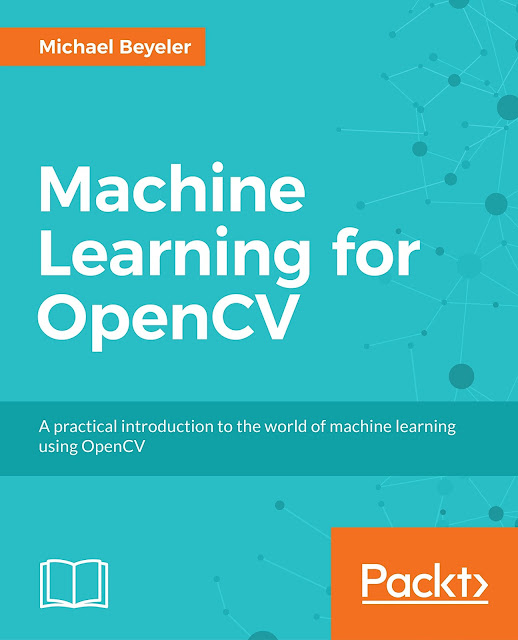## Monday, November 20, 2017

### How to integrate essential scikit-learn functions with OpenCV

OpenCV's machine learning module provides a lot of important estimators such as support vector machines (SVMs) or random forest classifiers, but it lacks scikit-learn-style utility functions for interacting with data, scoring a classifier, or performing grid search with cross-validation. In this post I will show you how to wrap an OpenCV classifier as a scikit-learn estimator in five simple steps so that you can still make use of scikit-learn utility functions when working with OpenCV.One of the advantages of wrapping OpenCV classifiers for scikit-learn is that you can now use the datasets module without having to worry about getting the data into the right format first:

In : from sklearn import datasets
...     iris = datasets.load_iris()


### Step 2: Wrapping the classifier

This is where all the magic happens. The main idea is to wrap an OpenCV classifier (e.g., cv2.ml.KNearest_create()) as a scikit-learn estimator. A scikit-learn estimator has the following properties:

• The class inherits from BaseEstimator, which is the base class of for all estimators in scikit-learn. In addition, the class can inherit from ClassifierMixin or RegressorMixin to receive a score method suitable for either classifiers or regressors:
In : import numpy as np
...     import cv2
...     import sklearn.base as sklb
...     class MyKnn(sklb.BaseEstimator,
...                 sklb.ClassifierMixin):
...         def __init__(self, k=1):
...             """An OpenCV-based k-nearest neighbor
...                classifier wrapped for scikit-learn
...
...             Parameters
...             ----------
...             k : int, optional, default: 1
...                 The number of neighbors to use by
...                 default.
...             """
...             self.k = k
...             self.knn = cv2.ml.KNearest_create()
...             self.knn.setDefaultK(k)

• The class requires a method get_params, which returns all arguments passed to the constructor in a dict:
...         def get_params(self, deep=True):
...             """Get parameters for this estimator"""
...             return {'k': self.k}

• The class requires a method set_params, which generates class attributes from all parameters in a dict, and returns self (note the documentation on this is wrong):
...         def set_params(self, **params):
...             """Set parameters for this estimator"""
...             for param, value in params.items():
...                 setattr(self, param, value)
...             return self

• The class requires a method fit, which fits the model to data (this is the same as the train method for OpenCV estimators) and returns self:
...         def fit(self, X, y):
...             """Fit the model using X as training
...                data and y as target values
...
...             Parameters
...             ----------
...             X : array of shape [n_samples,
...                                 n_features]
...                 Training data.
...             y : array of shape [n_samples]
...             """
...             self.knn.train(X.astype(np.float32),
...                            cv2.ml.ROW_SAMPLE, y)
...             return self

• The class requires a method predict, which predicts the target values for a set of provided data (equivalent to OpenCV's predict):
...         def predict(self, X):
...             """Predict the class labels for the
...                provided data
...
...             Parameters
...             ----------
...             X : array-like, shape (n_query,
...                                    n_features)
...                 Test samples.
...
...             Returns
...             -------
...             y : array of shape [n_samples] or
...                 [n_samples, n_outputs]
...                 Class labels for each data sample.
...             """
...             _, y_pred = self.knn.predict(
...                 X.astype(np.float32)
...             )
...             return y_pred


Note: You can use the same procedure to write any custom scikit-learn classifier—free from OpenCV.

### Step 3: Calling the classifier

With the above code you are now able to call and use the classifier in much the same way that you would use a scikit-learn estimator:

In : knn = MyKnn(3)
In : knn.fit(iris.data, iris.target)
Out: MyKnn(3)
In : knn.score(iris.data, iris.target)
Out: 0.95999999999999996


Note that the score method above was provided by the ClassifierMixin, which by default calculates the mean squared error between targets and predictions. Alternatively, you can specify your own scoring method that suits your need.

### Step 4: Using the classifier in cross-validation

In addition, you can now use scikit-learn's many utility functions, such as calculating the cross-validation score:

In : from sklearn.model_selection import cross_val_score
...     cross_val_score(MyKnn(3), iris.data, iris.target, cv=5)
Out: array([ 0.96666667,  0.96666667,  0.93333333,
0.96666667,  1.        ])


Here we are calculating the cross-validation scores across five folds for a k-NN classifier with k=3. The cross_val_score function automatically splits the data into train and test sets for each fold of the cross-validation procedure.

### Step 5: Using the classifier in grid search with cross-validation

Instead of writing these functions by hand, like you had to do when using with OpenCV classifiers, you can now perform procedures like grid search with cross-validation in only a few lines of code:

In : from sklearn.model_selection import GridSearchCV
...     grid = {'k': np.arange(1, 10)}
...     grid_search = GridSearchCV(MyKnn(), grid)
...     grid_search.fit(iris.data, iris.target)
Out: GridSearchCV(cv=None, error_score='raise',
estimator=MyKnn(k=1),
fit_params={}, iid=True, n_jobs=1,
param_grid={'k': array([1, 2, 3, 4, 5, 6, 7,
8, 9])},
pre_dispatch='2*n_jobs', refit=True,
return_train_score=True,
scoring=None, verbose=0)


Here we are searching for the k in range [1, 10) that gives us the lowest cross-validation error. The GridSearchCV object repeatedly performs cross-validation on the full iris dataset, and when done, allows us to find the best parameters and scores by accessing the grid_search instance:

In : grid_search.best_params_
Out: {'k': 1}
In : grid_search.best_score_
Out: 0.97333333333333338


You can find all of the above code in this handy GitHub Gist.For all things machine learning with OpenCV, check out my new book Machine Learning for OpenCV, Packt Publishing Ltd., July 2017.

As usual, all source code is available for free on GitHub.